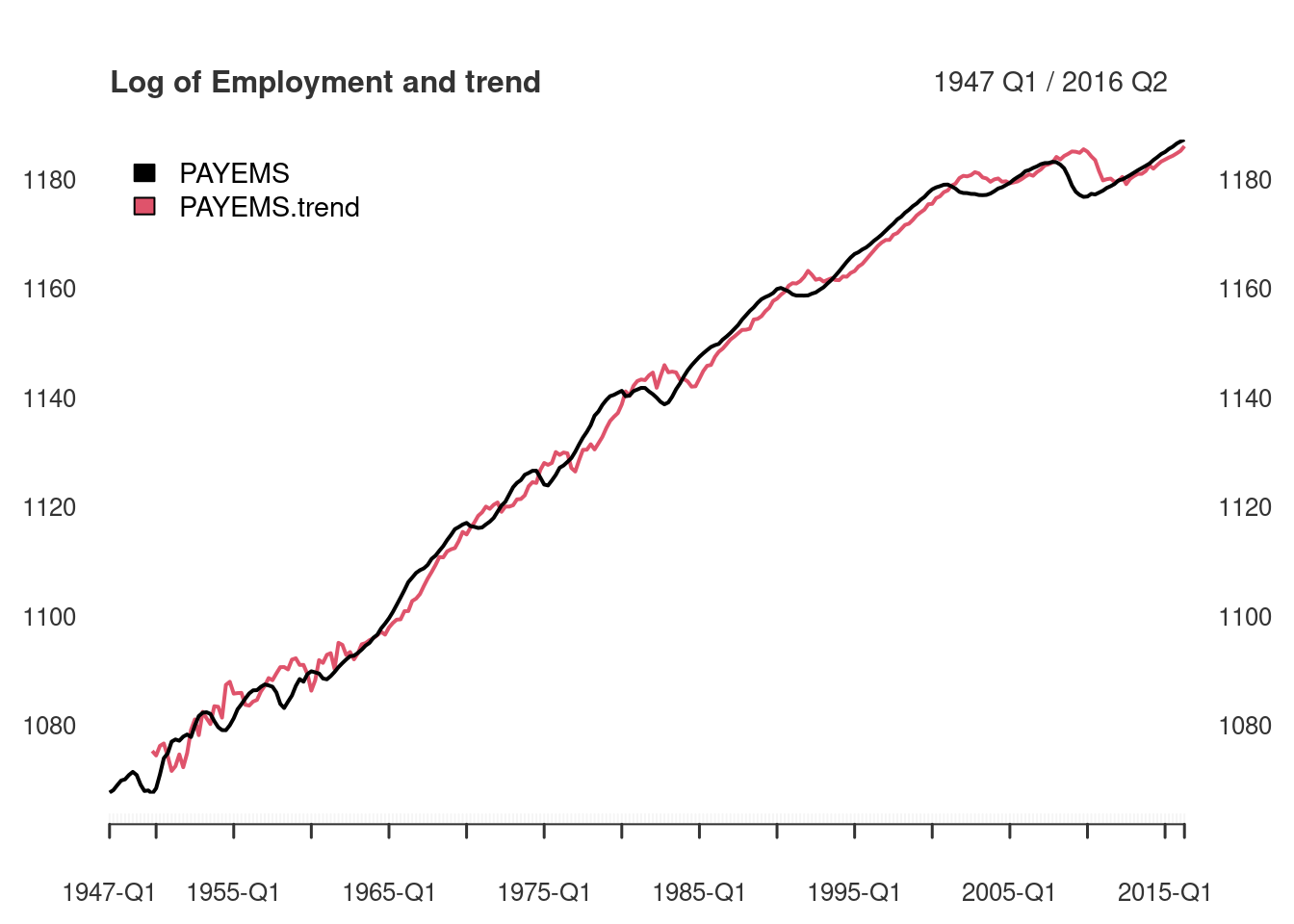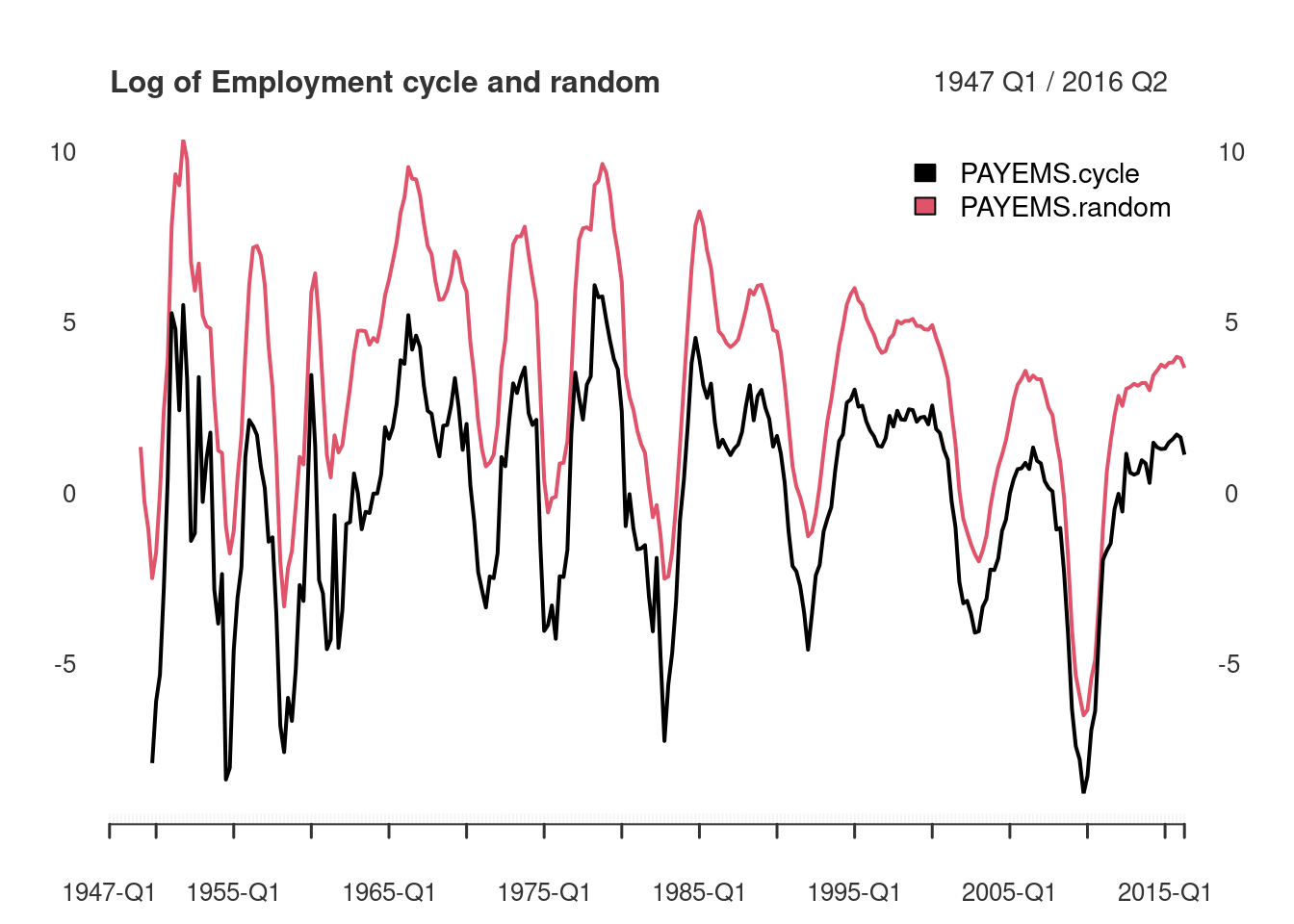In the working paper titled “Why You Should Never Use the Hodrick-Prescott Filter”, James D. Hamilton proposes an interesting new alternative to economic time series filtering. The neverhpfilter package provides functions for implementing his solution. Hamilton (2017) <doi:10.3386/w23429>

Hamilton’s abstract offers an excellent introduction to the problem and alternative solution:

1. The HP filter produces series with spurious dynamic relations that have no basis in the underlying data-generating process.
2. Filtered values at the end of the sample are very different from those in the middle, and are also characterized by spurious dynamics.
3. A statistical formalization of the problem typically produces values for the smoothing parameter vastly at odds with common practice, e.g., a value for $$\lambda$$ far below 1600 for quarterly data.
4. There’s a better alternative. A regression of the variable at date $$t + h$$ on the four most recent values as of date $$t$$ offers a robust approach to detrending that achieves all the objectives sought by users of the HP filter with none of its drawbacks.

Using quarterly economic data, Hamilton suggests a linear model on a univariate time series shifted ahead by h periods, regressed against a series of variables constructed from varying lags of the series by some number of periods, p. A modified auto-regressive $$AR(p)$$ model, dependent on a $$t+h$$ look-ahead, if you will. This is expressed more specifically by:

$y_{t+8} = \beta_0 + \beta_1 y_t + \beta_2 y_{t-1} +\beta_3 y_{t-2} + \beta_4 y_{t-3} + v_{t+8}$ $\hat{v}_{t+8} = y_{t+8} + \hat{\beta}_0 + \hat{\beta}_1 y_t + \hat{\beta}_2 y_{t-1} + \hat{\beta}_3 y_{t-2} + \hat{\beta}_4 y_{t-3}$

Which can be rewritten as:

$y_{t} = \beta_0 + \beta_1 y_{t-8} + \beta_2 y_{t-9} + \beta_3 y_{t-10} + \beta_4 y_{t-11} + v_{t}$

$\hat{v}_{t} = y_{t} - \hat{\beta}_0 + \hat{\beta}_1 y_{t-8} + \hat{\beta}_2 y_{t-9} + \hat{\beta}_3 y_{t-10} + \hat{\beta}_4 y_{t-11}$

## Implementation

First, lets run the yth_filter on Real GDP using the default settings suggested by Hamilton of an $$h = 8$$ look-ahead period (2 years) and $$p = 4$$ lags (1 year). The output is displayed below containing the original series, trend, cycle, and random components.

The random component is simply the difference between the original series and its $$h$$ look ahead, which is why it leads 8 NA observations. Due to the $$h$$ and $$p$$ parameters, trend and cycle components lead with 11 NA observations.

library(neverhpfilter)
data(GDPC1)

gdp_filter <- yth_filter(100*log(GDPC1), h = 8, p = 4)

head(data.frame(Date=index(gdp_filter), coredata(gdp_filter)), 15)
##       Date    GDPC1 GDPC1.trend GDPC1.cycle GDPC1.random
## 1  1947 Q1 761.7298          NA          NA           NA
## 2  1947 Q2 761.4627          NA          NA           NA
## 3  1947 Q3 761.2560          NA          NA           NA
## 4  1947 Q4 762.8081          NA          NA           NA
## 5  1948 Q1 764.3012          NA          NA           NA
## 6  1948 Q2 765.9384          NA          NA           NA
## 7  1948 Q3 766.5096          NA          NA           NA
## 8  1948 Q4 766.6213          NA          NA           NA
## 9  1949 Q1 765.2338          NA          NA     3.503988
## 10 1949 Q2 764.8921          NA          NA     3.429356
## 11 1949 Q3 765.9192          NA          NA     4.663188
## 12 1949 Q4 765.0764    772.5565  -7.4800757     2.268271
## 13 1950 Q1 768.9313    773.6959  -4.7646846     4.630074
## 14 1950 Q2 771.9355    774.7931  -2.8576273     5.997144
## 15 1950 Q3 775.7271    775.1513   0.5758054     9.217473

## Comparing our estimates with Hamilton’s

In this next section, I reproduce a few of Hamilton’s tables and graphs, to make sure the functions approximately match his results.

In the Appendix, Employment (All Employees: Total Non-farm series) is plotted in the form of $$100 * log($$PAYEMS$$)$$ and superimposed with it’s random walk representation. (Hamilton 44). There are many good reasons to use xts when handling time series data. Two of them are illustrated below in efficiently transforming monthly series to.quarterly and in ploting the results of yth_filter.

data(PAYEMS)
log_Employment <- 100*log(xts::to.quarterly(PAYEMS["1947/2016-6"], OHLC = FALSE))

employ_trend <- yth_filter(log_Employment, h = 8, p = 4, output = c("x", "trend"), family = gaussian)

plot.xts(employ_trend, grid.col = "white", legend.loc = "topleft", main = "Log of Employment and trend")When filtering time series, the cycle component is of great interest. Here, it is graphed alongside a random walk representation (Hamilton 44).

employ_cycle <- yth_filter(log_Employment, h = 8, p = 4, output = c("cycle", "random"), family = gaussian)

plot.xts(employ_cycle, grid.col = "white", legend.loc = "topright", main="Log of Employment cycle and random")
abline(h=0)Turning the page, we find a similar graph of the cyclical component of $$100 * log$$ of GDP, Exports, Consumption, Imports, Investment, and Government (Hamilton 45).

Below I merge these data into one xts object and write a function wrapper around yth_filter and plot, which is then lapply’d over each series, producing a plot for each one.

fig6_data <- 100*log(merge(GDPC1, EXPGSC1, PCECC96, IMPGSC1, GPDIC1, GCEC1)["1947/2016-3"])

fig6_wrapper <- function(x, ...) {
cycle <-  yth_filter(x, h = 8, p = 4, output = c("cycle", "random"), family = gaussian)
plot.xts(cycle, grid.col = "white", lwd=1, main = names(x))
}
par(mfrow=c(3,2))
lapply(fig6_data, fig6_wrapper)